# Azeotrope

﻿
Azeotrope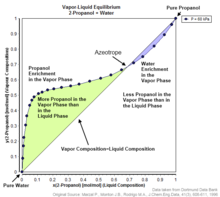Vapor-liquid equilibrium of 2-propanol/water showing azeotropic behavior

An azeotrope ( ə-zee-ə-trohp) is a mixture of two or more liquids in such a ratio that its composition cannot be changed by simple distillation. This occurs because, when an azeotrope is boiled, the resulting vapor has the same ratio of constituents as the original mixture.

Because their composition is unchanged by distillation, azeotropes are also called (especially in older texts) constant boiling mixtures. The word azeotrope is derived from the Greek words ζέειν (boil) and τρόπος (state) combined with the prefix α- (no) to give the overall meaning, “no change on boiling.”

Azeotropic mixtures of pairs of compounds have been documented. (See Azeotrope (data)). Many azeotropes of three or more compounds are also known.

## Types

### Positive and negative azeotropes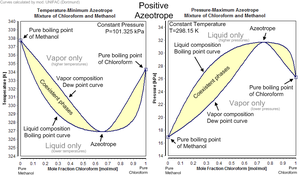Positive Azeotrope – Mixture of Chloroform and Methanol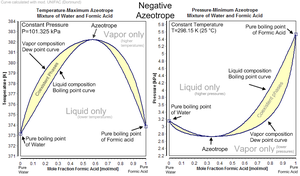Negative Azeotrope – Mixture of Formic acid and Water

Each azeotrope has a characteristic boiling point. The boiling point temperature of an azeotrope is either less than the boiling point temperatures of any of its constituents (a positive azeotrope), or greater than the boiling point temperatures of any of its constituents (a negative azeotrope).

A well known example of a positive azeotrope is 95.63% ethanol and 4.37% water (by weight). Ethanol boils at 78.4°C, water boils at 100°C, but the azeotrope boils at 78.2°C, which is lower than either of its constituents. Indeed 78.2°C is the minimum temperature at which any ethanol/water solution can boil at atmospheric pressure. In general, a positive azeotrope boils at a lower temperature than any other ratio of its constituents. Positive azeotropes are also called minimum boiling mixtures or pressure maximum azeotropes.

An example of a negative azeotrope is hydrochloric acid at a concentration of 20.2% and 79.8% water (by weight). Hydrogen chloride boils at −84°C and water at 100°C, but the azeotrope boils at 110°C, which is higher than either of its constituents. The maximum temperature at which any hydrochloric acid solution can boil is 110°C. In general, a negative azeotrope boils at a higher temperature than any other ratio of its constituents. Negative azeotropes are also called maximum boiling mixtures or pressure minimum azeotropes.

### Homogeneous and heterogeneous azeotropes

If the constituents of a mixture are not completely miscible an azeotrope can be found inside the miscibility gap. This type of azeotrope is called heterogeneous azeotrope. If the azeotropic composition is outside the miscibility gap or the constituents of the mixture are completely miscible the type of azeotrope is called a homogeneous azeotrope.

### Number of constituents

Azeotropes consisting of two constituents, such as the two examples above, are called binary azeotropes. Those consisting of three constituents are called ternary azeotropes. Azeotropes of more than three constituents are also known.

### Zeotropy

Combinations of solvents that do not form an azeotrope when mixed in any proportion are said to be zeotropic.

## Distillation of mixtures

If two solvents can form a positive azeotrope, then distillation of any mixture of those constituents will result in the distillate being closer in composition to the azeotrope than the starting mixture. For example, if a 50/50 mixture of ethanol and water is distilled once, the distillate will be 80% ethanol and 20% water (see ethanol data page), which is closer to the azeotropic mixture than the original. Distilling the 80/20% mixture produces a distillate that is 87% ethanol and 13% water. Further repeated distillations will produce mixtures that are progressively closer to the azeotropic ratio of 95.5/4.5%. No number of distillations, however, will ever result in a distillate that exceeds the azeotropic ratio. Likewise when distilling a mixture of ethanol and water that is richer in ethanol than the azeotrope, the distillate (contrary to intuition) will be poorer in ethanol than the original but slightly richer than the azeotrope. This means the solution left behind will be richer in ethanol.

If two solvents can form a negative azeotrope, then distillation of any mixture of those constituents will result in the residue being closer in composition to the azeotrope than the original mixture. For example, if a hydrochloric acid solution contains less than 20.2% hydrogen chloride, boiling the mixture will leave behind a solution that is richer in hydrogen chloride than the original. If the solution initially contains more than 20.2% hydrogen chloride, then boiling will leave behind a solution that is poorer in hydrogen chloride than the original. Boiling of any hydrochloric acid solution long enough will cause the solution left behind to approach the azeotropic ratio.

## Phase diagrams

The boiling and recondensation of a mixture of two solvents are changes of state. As such, they are best illustrated with a phase diagram. If pressure is held constant, the two parameters that can vary are the temperature and the composition. An azeotrope is not the same as an emulsion.

### Minimum-boiling or Positive azeotrope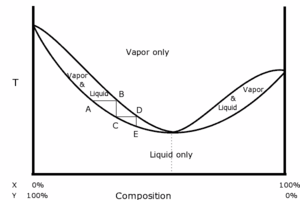Phase diagram of a positive azeotrope. Vertical axis is temperature, horizontal axis is composition.

The diagram on the right shows a positive azeotrope of hypothetical constituents, X and Y. The bottom trace illustrates the boiling temperature of various compositions. Below the bottom trace, only the liquid phase is in equilibrium. The top trace illustrates the vapor composition above the liquid at a given temperature. Above the top trace, only the vapor is in equilibrium. Between the two traces, liquid and vapor phases exist simultaneously in equilibrium: for example, heating a 25% X : 75% Y mixture to temperature AB would generate vapor of composition B over liquid of composition A. The azeotrope is the point on the diagram where the two curves touch. The horizontal and vertical steps show the path of repeated distillations. Point A is the boiling point of a nonazeotropic mixture. The vapor that separates at that temperature has composition B. The shape of the curves requires that the vapor at B be richer in constituent X than the liquid at point A. The vapor is physically separated from the VLE (vapor-liquid equilibrium) system and is cooled to point C, where it condenses. The resulting liquid (point C) is now richer in X than it was at point A. If the collected liquid is boiled again, it progresses to point D, and so on. The stepwise progression shows how repeated distillation can never produce a distillate that is richer in constituent X than the azeotrope. Note that starting to the right of the azeotrope point results in the same stepwise process closing in on the azeotrope point from the other direction.

### Maximum-boiling or Negative azeotrope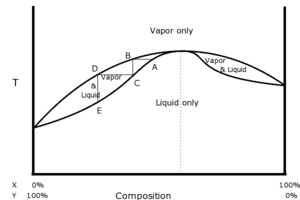Phase diagram of a negative azeotrope. Vertical axis is temperature, horizontal axis is composition.

The diagram on the right shows a negative azeotrope of hypothetical constituents, X and Y. Again the bottom trace illustrates the boiling temperature at various compositions, and again, below the bottom trace the mixture must be entirely liquid phase. The top trace again illustrates the condensation temperature of various compositions, and again, above the top trace the mixture must be entirely vapor phase. The point, A, shown here is a boiling point with a composition chosen very near to the azeotrope. The vapor is collected at the same temperature at point B. That vapor is cooled, condensed, and collected at point C. Because this example is a negative azeotrope rather than a positive one, the distillate is farther from the azeotrope than the original liquid mixture at point A was. So the distillate is poorer in constituent X and richer in constituent Y than the original mixture. Because this process has removed a greater fraction of Y from the liquid than it had originally, the residue must be poorer in Y and richer in X after distillation than before.

If the point, A, had been chosen to the right of the azeotrope rather than to the left, the distillate at point C would be farther to the right than A, which is to say that the distillate would be richer in X and poorer in Y than the original mixture. So in this case too, the distillate moves away from the azeotrope and the residue moves toward it. This is characteristic of negative azeotropes. No amount of distillation, however, can make either the distillate or the residue arrive on the opposite side of the azeotrope from the original mixture. This is characteristic of all azeotropes.

### Why there are two traces

The traces in the phase diagrams separate whenever the composition of the vapor differs from the composition of the liquid at the same temperature. Suppose the total composition were 50/50%. You could make this composition using 50% of 50/50% vapor and 50% of 50/50% liquid, but you could also make it from 83.33% of 45/55% vapor and 16.67% of 75%/25% liquid, as well as from many other combinations. The separation of the two traces represents the range of combinations of liquid and vapor that can make each total composition.

Alternatively, one can view the lower trace as the boundary for the region of the diagram in which liquids are in equilibrium, and the upper trace as the boundary of the region in which the vapor is in equilibrium. These two boundaries need not coincide. Indeed, the region between them is a no-man's-land: attempts to bring the system to the midpoint of line-segment AB will result in a mixture of liquid A and vapor B, but nothing at the midpoint.

## Heteroazeotropes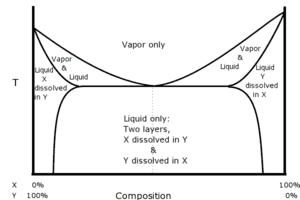Phase diagram of a heteroazeotrope. Vertical axis is temperature, horizontal axis is composition. The dotted vertical line indicates the composition of the combined layers of the distillate whenever both layers are present in the original mixture.

In each of the examples discussed so far the constituents have been miscible in all proportions with each other. For example, any amount of ethanol can be mixed with any amount of water to form a homogeneous solution. There are pairs of solvents for which this is not the case. For example, if equal volumes of chloroform (water solubility 0.8 g/100 ml at 20°C) and water are shaken together and then left to stand, the liquid will separate into two layers. Analysis of the layers shows that the top layer is mostly water with a small amount of chloroform dissolved in it, and the bottom layer is mostly chloroform with a small amount of water dissolved in it. If the two layers are heated together, the system of layers will boil at 53.3°C, which is lower than either the boiling point of chloroform (61.2°C) or the boiling point of water (100°C). The vapor will consist of 97.0% chloroform and 3.0% water regardless of how much of each liquid layer is present (provided both layers are indeed present). If the vapor is re-condensed, the layers will reform in the condensate, and will do so in a fixed ratio, which in this case is 4.4% of the volume in the top layer and 95.6% in the bottom layer. Such a system of solvents is known as a heteroazeotrope. The diagram illustrates how the various phases of a heteroazeotrope are related.

Heteroazeotropes are always minimum boiling mixtures.

## Deviation from Raoult's law

Raoult's law predicts the vapor pressures of ideal mixtures as a function of composition ratio. In general only mixtures of chemically similar solvents, such as n-hexane with n-heptane, form nearly ideal mixtures that come close to obeying Raoult's law. Solvent combinations that can form azeotropes are always nonideal, and as such they deviate from Raoult's law.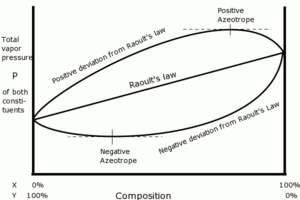Total vapor pressure of mixtures as a function of composition at a chosen constant temperature.

The diagram on the right illustrates total vapor pressure of three hypothetical mixtures of constituents, X, and Y. The temperature throughout the plot is assumed to be constant.

The center trace is a straight line, which is what Raoult's law predicts for an ideal mixture. The top trace illustrates a nonideal mixture that has a positive deviation from Raoult's law, where the total combined vapor pressure of constituents, X and Y, is greater than what is predicted by Raoult's law. The top trace deviates sufficiently that there is a point on the curve where its tangent is horizontal. Whenever a mixture has a positive deviation and has a point at which the tangent is horizontal, the composition at that point is a positive azeotrope. At that point the total vapor pressure is at a maximum. Likewise the bottom trace illustrates a nonideal mixture that has a negative deviation from Raoult's law, and at the composition where tangent to the trace is horizontal there is a negative azeotrope. This is also the point where total vapor pressure is minimum.

### Temperature-pressure dependence

For both the top and bottom traces, the temperature point of the azeotrope is the constant temperature chosen for the graph. If the ambient pressure is controlled to be equal to the total vapor pressure at the azeotropic mixture, then the mixture will boil at this fixed temperature.

Vapor pressure of both pure liquids as well as mixtures is a sensitive function of temperature. As a rule, vapor pressure of a liquid increases nearly exponentially as a function of temperature. If the graph were replotted for a different fixed temperature, then the total vapor pressure at the azeotropic composition will certainly change, but it is also possible that the composition at which the azeotrope occurs will change. This implies that the composition of an azeotrope is affected by the pressure chosen at which to boil the mixture. Ordinarily distillation is done at atmospheric pressure, but with proper equipment it is possible to carry out distillation at a wide variety of pressures, both above and below atmospheric pressure.

## Separation of azeotrope constituents

Distillation is one of the primary tools that chemists and chemical engineers use to separate mixtures into their constituents. Because distillation cannot separate the constituents of an azeotrope, the separation of azeotropic mixtures (also called azeotrope breaking) is a topic of considerable interest. Indeed this difficulty led some early investigators to believe that azeotropes were actually compounds of their constituents. But there are two reasons for believing that this is not the case. One is that the molar ratio of the constituents of an azeotrope is not generally the ratio of small integers. For example, the azeotrope formed by water and acetonitrile contains 2.253 moles of acetonitrile for each mole of water. A more compelling reason for believing that azeotropes are not compounds is, as discussed in the last section, that the composition of an azeotrope can be affected by pressure. Contrast that with a true compound, carbon dioxide for example, which is two moles of oxygen for each mole of carbon no matter what pressure the gas is observed at. That azeotropic composition can be affected by pressure suggests a means by which such a mixture can be separated.

### Pressure swing distillation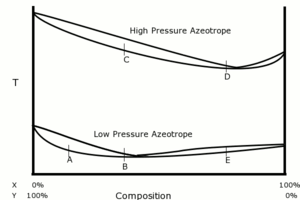Azeotrope composition shift due to pressure swing.

A hypothetical azeotrope of constituents X and Y is shown in the diagram to the right. Two plots are shown, one at an arbitrary low pressure and another at an equally arbitrary, but higher, pressure. The composition of the azeotrope is substantially different between the high and low pressure plots – higher in X for the high-pressure system. The goal is to separate X in as high a concentration as possible starting from point A. At the low pressure, it is possible by progressive distillation to reach a distillate at the point, B, which is on the same side of the azeotrope as A. Note that successive distillation steps near the azeotropic composition exhibit very little difference in boiling temperature. If this distillate is now exposed to the high pressure, it boils at point C. From C, by progressive distillation it is possible to reach a distillate at the point D, which is on the same side of the high pressure azeotrope as C. If that distillate is then exposed again to the low pressure, it boils at point E, which is on the opposite side of the low pressure azeotrope to A. So, by means of the pressure swing, it was possible to cross over the low pressure azeotrope.

When the solution is boiled at point E, the distillate is poorer in X than point E. This means that the residue is made richer in X than point E. Indeed, progressive distillation can result in a residue that is as rich in X as is required.

In summary:

 1. Low pressure rectification (A to B) 2. High pressure rectification (C to D) 3. Low pressure stripping (E to target purity) Rectification: the distillate, or "tops", is retained and exhibits an increasingly lower boiling point. Stripping: the residue, or "bottoms", is retained and exhibits an increasingly higher boiling point.

Note that both azeotropes above are of the positive, or minimum boiling type; care must be taken to ensure that the correct component of the separation step is retained, i.e. the binary phase-envelope diagram (boiling-point curve) must be correctly read.

A mixture of 5% water with 95% tetrahydrofuran is an example of an azeotrope that can be economically separated using a pressure swing – a swing in this case between 1 atm and 8 atm. By contrast the composition of the water/ethanol azeotrope discussed earlier is not affected enough by pressure to be easily separated using pressure swings and instead, an entrainer may be added that either modifies the azeotropic composition and exhibits immiscibility with one of the components, or extractive distillation may be used.

### Azeotropic distillation

Other methods of separation involve introducing an additional agent, called an entrainer, that will affect the volatility of one of the azeotrope constituents more than another. When an entrainer is added to a binary azeotrope to form a ternary azeotrope, and the resulting mixture distilled, the method is called azeotropic distillation. The best known example is adding benzene or cyclohexane to the water/ethanol azeotrope. With cyclohexane as the entrainer, the ternary azeotrope is 7% water, 17% ethanol, and 76% cyclohexane, and boils at 62.1°C. Just enough cyclohexane is added to the water/ethanol azeotrope to engage all of the water into the ternary azeotrope. When the mixture is then boiled, the azeotrope vaporizes leaving a residue composed almost entirely of the excess ethanol.

### Chemical action separation

Another type of entrainer is one that has a strong chemical affinity for one of the constituents. Using again the example of the water/ethanol azeotrope, the liquid can be shaken with calcium oxide, which reacts strongly with water to form the nonvolatile compound, calcium hydroxide. Nearly all of the calcium hydroxide can be separated by filtration and the filtrate redistilled to obtain 100% pure ethanol.

A more extreme example is the azeotrope of 1.2% water with 98.8% diethyl ether. Ether holds the last bit of water so tenaciously that only a very powerful desiccant such as sodium metal added to the liquid phase can result in completely dry ether.

Anhydrous calcium chloride is used as a desiccant for drying a wide variety of solvents since it is inexpensive and does not react with most nonaqueous solvents. Chloroform is an example of a solvent that can be effectively dried using calcium chloride.

### Distillation using a dissolved salt

When a salt is dissolved in a solvent, it always has the effect of raising the boiling point of that solvent – that is it decreases the volatility of the solvent. When the salt is readily soluble in one constituent of a mixture but not in another, the volatility of the constituent in which it is soluble is decreased and the other constituent is unaffected. In this way, for example, it is possible to break the water/ethanol azeotrope by dissolving potassium acetate in it and distilling the result.

### Extractive distillation

Extractive distillation is similar to azeotropic distillation, except in this case the entrainer is less volatile than any of the azeotrope's constituents. For example, the azeotrope of 20% acetone with 80% chloroform can be broken by adding water and distilling the result. The water forms a separate layer in which the acetone preferentially dissolves. The result is that the distillate is richer in chloroform than the original azeotrope.

### Pervaporation and other membrane methods

The pervaporation method uses a membrane that is more permeable to the one constituent than to the another to separate the constituents of an azeotrope as it passes from liquid to vapor phase. The membrane is rigged to lie between the liquid and vapor phases. Another membrane method is vapor permeation, where the constituents pass through the membrane entirely in the vapor phase. In all membrane methods, the membrane separates the fluid passing through it into a permeate (that which passes through) and a retentate (that which is left behind). When the membrane is chosen so that is it more permeable to one constituent than another, then the permeate will be richer in that first constituent than the retentate.

## Use of azeotropes to separate azeotropic mixtures

Sometimes azeotropes are useful in separating zeotropic mixtures. An example is acetic acid and water, which do not form an azeotrope. Despite this it is very difficult to separate pure acetic acid (boiling point: 118.1°C) from a solution of acetic acid and water by distillation alone. As progressive distillations produce solutions with less and less water, each further distillation becomes less effective at removing the remaining water. Distilling the solution to dry acetic acid is therefore economically impractical. But ethyl acetate forms an azeotrope with water that boils at 70.4°C. By adding ethyl acetate as an entrainer, it is possible to distill away the azeotrope and leave nearly pure acetic acid as the residue.

## Why azeotropes exist

Azeotropes can only form when a mixture deviates from Raoult's law. Raoult's law applies when the molecules of the constituents stick to each other to the same degree as they do to themselves. For example, if the constituents are X and Y, then X sticks to Y with roughly equal energy as X does with X and Y does with Y. A positive deviation from Raoult's law results when the constituents have a disaffinity for each other – that is X sticks to X and Y to Y better than X sticks to Y. Because this results in the mixture having less total sticking together of the molecules than the pure constituents, they more readily escape from the stuck-together phase, which is to say the liquid phase, and into the vapor phase. When X sticks to Y more aggressively than X does to X and Y does to Y, the result is a negative deviation from Raoult's law. In this case because there is more sticking together of the molecules in the mixture than in the pure constituents, they are more reluctant to escape the stuck-together liquid phase.

When the deviation is great enough to cause a maximum or minimum in the vapor pressure versus composition function, it is a mathematical consequence that at that point, the vapor will have the same composition as the liquid, and so an azeotrope is the result.

## Complex azeotrope systems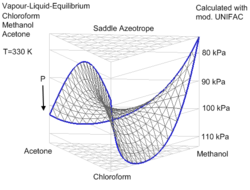Saddle azeotropic system Methanol/Acetone/Chloroform calculated with mod. UNIFAC

The rules for positive and negative azeotropes apply to all the examples discussed so far. But there are some examples that don't fit into the categories of positive or negative azeotropes. The best known of these is the ternary azeotrope formed by 30% acetone, 47% chloroform, and 23% methanol, which boils at 57.5°C. Each pair of these constituents forms a binary azeotrope, but chloroform/methanol and acetone/methanol both form positive azeotropes while chloroform/acetone forms a negative azeotrope. The resulting ternary azeotrope is neither positive nor negative. Its boiling point falls between the boiling points of acetone and chloroform, so it is neither a maximum nor a minimum boiling point. This type of system is called a saddle azeotrope. Only systems of three or more constituents can form saddle azeotropes.

A rare type of complex binary azeotrope is one where the boiling point and condensation point curves touch at two points in the phase diagram. Such a system is called a double azeotrope, and will have two azeotropic compositions and boiling points. An example is water and N-methylethylenediamine.

## Examples of azeotropes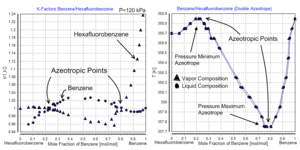Double azeotrope of benzene and hexafluorobenzene, taken from Dortmund Data Bank

Proportions are by weight.